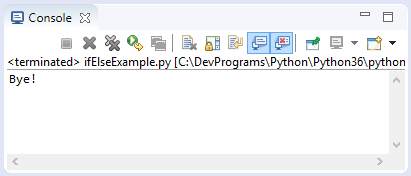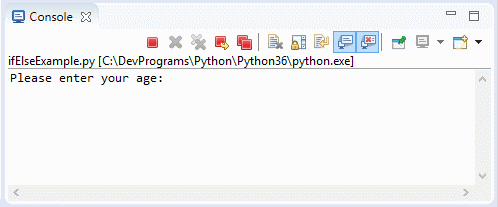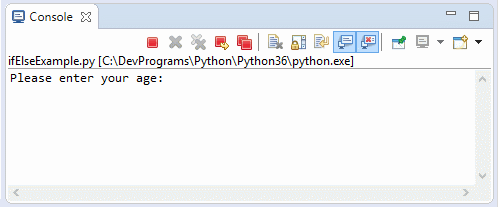# Branching statements in Python

## 1- Comparison operators

The common comparison operators:
 Operator Meaning Example > greater than 5 > 4 is true < less than 4 < 5 is true >= greater than or equal 4 >= 4 is true <= less than or equal 3 <= 4 is true == equal to 1 == 1 is true != not equal to 1 != 2 is true and and a > 4 and a < 10 or or a == 1 or a == 4

## 2- if-else statement

if is a statement which checks  a certain condition in Python. For example: If a> b, then do something ....

Syntax:
```if condition_1 :
# Do something

elif condition_2 :
# Do something

elif condition_N:
# Do something

else :
# Do something

```
The program checks the condition from top to bottom until it encounters a condition that is true, and run this block and the program does not check the remaining conditions in the branching structure.
Example (if - else):
ifElseExample.py
```option = 5

if option == 1:

print("Hello")

else :

print("Bye!")
```Example (if - elif - else):
ifElseExample2.py
```print("Please enter your age: \n")

# Declare a variable to store the user input from the keyboard.
inputStr =  input()

# int(..) function convert string to integer
age = int(inputStr)

# If age < 80 then ..
if (age < 80) :

print("You are pretty young")

# Else if age between 80, 100 then
elif (age >= 80 and age <= 100) :

print("You are old")

# Else (Other case)
else :

print("You are verry old")
```
Running the example:#### View more Tutorials:

Maybe you are interested

These are online courses outside the o7planning website that we introduced, which may include free or discounted courses.

•Complete Python Web Course: Build 8 Python Web Apps
•Learn Programming in Python With the Power of Animation
•Python 3 For Beginner - Object-Oriented Programming
•Python Fundamentals
•Learning Path: Python GUI Projects
•Python BeautifulSoup: Extract Web Data Beautifully
•Master Computer Vision™ OpenCV4 in Python with Deep Learning
•Python Programming Full Course (Basics,OOP,Modules,PyQt)
•Python 2000: Beyond The Basics
•Web Programming with Python
•Learning Path: Python Web Development
•Learn iPython: The Full Python IDE
•New to Python Automation..?Try Step by Step Python 4 Testers
•Python GUI : From A-to-Z With 2 Final Projects
•Python 3000: Tactical SQL Quick-Start